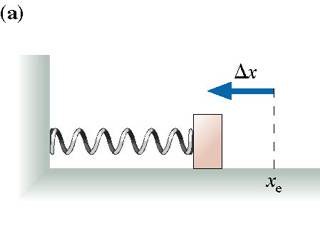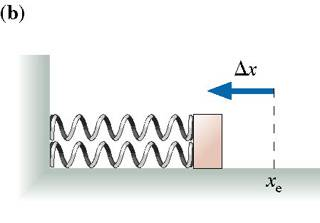hey guys, how would i go about solving this?

The spring in the figure (a) is compressed by length x. It launches the block across a frictionless surface with speed v_0. The two springs in the figure (b) are identical to the spring of the figure (a). They are compressed by the same length Deltax and used to launch the same block.What is the block's speed?i know that this has to do something with v_0 raised to a power, but i cant figure out which power. please help

Last edited:

I guess you'll need to use the conservation of energy (Etotal)before the motion= (Etotal)after the motion.

Think carefully: at the initial situation of pic A, what energy does the block contain (i.e. does it have any kinetic energy?, or potential energy?). Write down formulas for the types of energy the block has (i.e. kinetic energy= $$\frac{1}{2} mv^2$$ and add them. Now take a closer look at the variables in the formula:which values do they have in this situation?

Next consider the types of energies the block has when it has just left the spring and repeat the steps you took when you found an expression for the energy the block had at the initial situation. Be sure to equal both obtained equations and use it to obtain an expression for $$v_0$$.

Then consider situation B and do the same things you've done for situation A in order to obtain a different equation, but be sure to take into consideration that now there are two springs pushing the block, so instead of the spring constant C in part A you now are dealing with 2C. The equation you get from analyzing situation B can be written down in a way so you get an expression for v.

Now you only have to take a look at the expression of $$v_0$$ and compare it to the one you have for v.

Good luck! And if you don't understand some of my explanation, don't hesitate and please ask!

EDIT: :P Just found out you've already solved the problem ;)

Last edited: